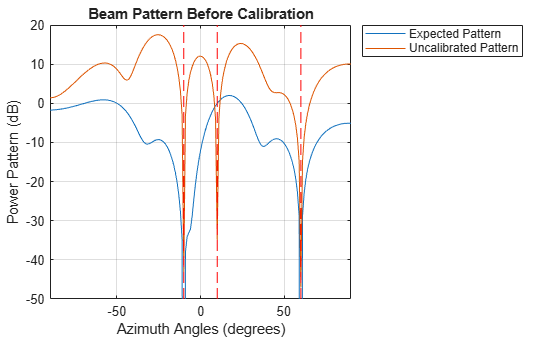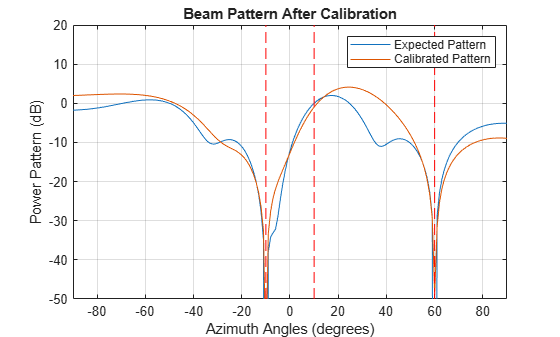Using Pilot Calibration to Compensate For Array Uncertainties

This example shows how to use pilot calibration to improve the performance of an antenna array in the presence of unknown perturbations.

Introduction

In principle, one can easily design an ideal uniform linear array (ULA) to perform array processing tasks such as beamforming or direction of arrival estimation. In practice, there is no such thing as an ideal array. For example, there will always be some inevitable manufacturing tolerances among different elements within the array. Since in general it is impossible to obtain exact knowledge about those variations, they are often referred to as uncertainties or perturbations. Commonly observed uncertainties include element gain and element phase uncertainties (electrical uncertainties) as well as element location uncertainties (geometrical uncertainties).

The presence of uncertainties in an array system causes rapid degradation in the detection, resolution, and estimation performance of array processing algorithms. Therefore it is critical to calibrate the array before its deployment. In addition to the aforementioned factors, uncertainties can also arise due to other factors such as hardware aging and environmental effects. Calibration is therefore also performed on a regular basis in all deployed systems.

There are many array calibration algorithms. This example focuses on the pilot calibration approach , where the uncertainties are estimated from the response of the array to one or more known external sources at known locations. The example compares the effect of uncertainties on the array performance before and after the calibration.

Modeling Electrical and Geometrical Uncertainties

Consider an ideal 6-element ULA along y-axis operating with half-wavelength spacing and uniform tapering. For a ULA, the expected element positions and tapers can be computed.

N = 6;
designed_pos = [zeros(1,N);(0:N-1)*0.5;zeros(1,N)];
designed_taper = ones(N,1);

Next, model the perturbations that may exist in a real array. These are usually modeled as random variables. For example, assume that the taper's magnitude and phase are perturbed by normally distributed random variables with standard deviations of 0.1 and 0.05, respectively.

rng(2014);
taper = (designed_taper + 0.1*randn(N,1)).*exp(1i*0.05*randn(N,1));

The following figure shows the difference between the magnitude and phase of the perturbed taper and the designed taper.

helperCompareArrayProperties('Taper',taper,designed_taper,...
{'Perturbed Array','Designed Array'});Perturbations in the sensor locations in the x, y, and z directions are generated similarly with a standard deviation of 0.05.

pos = designed_pos + 0.05*randn(3,N);

The figure below shows where the element positions of the perturbed array and the ideal array.

helperCompareArrayProperties('Position',pos,designed_pos,...
{'Perturbed Array','Designed Array'});Effect of Array Perturbation

The previous section shows the difference between the designed, ideal array and the real, perturbed array. Because of these errors, if one blindly applies processing steps, such as beamforming weights, computed using the designed array, on the perturbed array, the performance degrades significantly.

Consider the case of an LCMV beamformer designed to steer the ideal array to a direction of 10 degrees azimuth with two interferences from two known directions of -10 degrees azimuth and 60 degrees azimuth. The goal is to preserve the signal of interest while suppressing the interferences.

If the precise knowledge of the array's taper and geometry is known, the beamforming weights can be computed as follows:

% Generate 10K samples from target and interferences with 30dB SNR
az = [-10 10 60];
Nsamp = 1e4;
ncov = db2pow(-30);
[~,~,rx_cov] = sensorsig(pos,Nsamp,az,ncov,'Taper',taper);

% Compute LCMV beamforming weights assuming the designed array
sv = steervec(pos,az);
w = lcmvweights(bsxfun(@times,taper,sv),[0;1;0],rx_cov);

However, since the array contains unknown perturbations, beamforming weights must be computed based on the positions and taper of the designed array.

designed_sv = steervec(designed_pos,az);
designed_w = lcmvweights(bsxfun(@times,designed_taper,designed_sv),...
[0;1;0],rx_cov);

The following figure compares the expected beam pattern with the pattern resulted from applying the designed weights on the perturbed array.

helperCompareBeamPattern(pos,taper,w,designed_w,-90:90,az,...
{'Expected Pattern','Uncalibrated Pattern'},...
'Beam Pattern Before Calibration');From the plotted patterns, it is clear that the pattern resulted from the uncalibrated weights does not satisfy the requirements. It puts a null around the desired 10 degrees azimuth direction. This means that the desired signal can no longer be retrieved. Fortunately, array calibration can help bring the pattern back to order.

Pilot Calibration

There are many algorithms available to perform array calibration. One class of commonly used algorithms is pilot calibration. The algorithm sets up several sources in known directions and then uses the array to receive the signal from those transmitters. Because these transmitters are at the known directions, the expected received signal of the ideal array can be computed. Comparing these with the actual received signal, it is possible to derive the difference due to the uncertainties and correct them.

The code below shows the process of array calibration. First, the pilot sources need to be chosen at different directions. Note that the number of pilot sources determines how many uncertainties the algorithm can correct. In this example, to correct both sensor location uncertainties and taper uncertainty, a minimum of four external sources is required. If more sources are used, the estimation will improve.

pilot_ang = [-60, -5 ,5, 40; -10, 0, 0, 30];

The four pilot sources are located at the following azimuth and elevation angle pairs: (-60, -10), (-5, 0), (5, 0), and (40, 30). The received signal from these pilots can be simulated as

for m = size(pilot_ang,2):-1:1
calib_sig(:,:,m) = sensorsig(pos,Nsamp,pilot_ang(:,m),...
ncov,'Taper',taper);
end

Using the received signal from the pilots at the array, together with the element positions and tapers of the designed array, the calibration algorithm  estimates the element positions and tapers for the perturbed array.

[est_pos,est_taper] = pilotcalib(designed_pos,...
calib_sig,pilot_ang,designed_taper);

Once the estimated positions and taper are available, these can be used in place of the designed array parameters when calculating beamformer weights. This results in the array pattern represented by the red line below.

corrected_w = lcmvweights(bsxfun(@times,est_taper,...
steervec(est_pos,az)),[0;1;0],rx_cov);

helperCompareBeamPattern(pos,taper,...
w,corrected_w,-90:90,az,...
{'Expected Pattern','Calibrated Pattern'},...
'Beam Pattern After Calibration');The figure above shows that the pattern resulting from the calibrated array is much better than the one from the uncalibrated array. In particular, signals from the desired direction are now preserved.

Summary

This example shows how uncertainties of an array can impact its response pattern and in turn degrade the array's performance. The example also illustrates how pilot calibration can be used to help restore the array performance.

References

 N. Fistas and A. Manikas, "A New General Global Array Calibration Method", IEEE Proceedings of ICASSP, Vol. IV, pp. 73-76, April 1994.